# Superposition principle — in layman’s terms

While Schrödinger’s cat illustrates superposition, it may trigger some simple questions

• When we physically open the box, there is a 50% chance of seeing the cat either live or dead (clearly not both). So what does it even mean to say the cat is both live and dead when we haven’t looked inside, apart from the question evoking memory of equivalently vacuous babble on the same topic about trees falling in forests and not being heard etc?

The following game will show how we can increase the odds of winning from 50% with a regular fair coin to 100% with a “quantum coin” by

• leveraging off information equivalent to “both live and dead cat state”, that is the quantum coin “in both heads and tails state” — and just a little cheating.

Regular coin game.

Lets first do the game with a regular fair coin.

1. We place coin in a box.

It is obvious that our chances of winning is 50%. Also, it doesn’t really matter if we opened the box and looked inside at step 4. If we played this game, say a 1000 times, regardless of us looking at the intermediate result at step 4, the chance of winning is always 50%. Figure 1 illustrates this.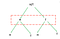Figure 1. Regular coin toss outcomes. The first toss yields heads/tails with 50% chance each. Subsequent tosses yields heads/tails with 50% chance. So chances of winning after second toss is still 50%. It doesn’t matter if we open the box after the first toss — the chances remain unchanged regardless of observing coin after first toss (observation indicated in red)

Quantum coin game.

Lets now play the game with a quantum coin in the box. The quantum coin is similar to the regular coin — meaning it can be in two states, head or tail, and there is a 50% chance of it being in either of these states after an operation equivalent to shaking the box.

1. We place quantum coin in a box.

We can repeat this game any number of times, and so long we cheat each time and our opponent doesn’t notice, we can win. Lets say, opponent notices that we are always looking at the quantum coin before the first coin toss.

• The opponent may suspect the quantum coin is not a fair coin like the regular one, and may ask us to open the box after the first shake in step 4.

So even though the opponent knows our looking into the quantum box before first shake has something to do with winning, it will not make sense how that can enable us to win, because opening the box after first shake showed the quantum coin behaved exactly like a fair coin.

We can now let in the opponent on our secret. We can confess even we don’t understand it too from a common sense perspective, except the following strategy always works

• if we see the quantum coin before first toss, we can always win so long as we don’t open the box after the first shake. Figure 2 illustrates this for head case and Figure 3 for tail case start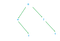Figure 2. Quantum coin toss outcome always yields a tail after second shake when we start off with a head and do not open the box and observe coin after first shake.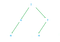Figure 3. Quantum coin toss outcome always yields a head after second shake when we start off with a tail and do not open the box and observe coin after first shake.
• We can also say, our chances of winning with a quantum coin becomes exactly like a regular coin toss once we open after first shake and observe its state, which is precisely why we didn’t bet when opponent asked us to open the box after first shake. This is shown in figure 4 below.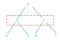Figure 4. Quantum coin toss outcome behaves exactly like a regular coin, regardless of starting off with head or tails and we open the box and observe coin after first shake. The odds of winning is now same as regular coin — 50%.Red box indicates looking at outcome after first shake

So to summarize the strangeness of the quantum coin toss

• Observing the quantum coin after first shake, clearly shows it is like a fair coin. We can even count the four paths from the root leading to the four outcomes regardless of what we start with at root — HH,HT,TH,TT — all occur with equal chances leading to 50% each for head and tail on second shake.

The mathematical explanation (without equations) for how two of the four branches disappear is quite simple.

• When it comes to quantum stuff, like quantum coins, we change the rules of calculating probability and allow for the paths of the tree in the figures for quantum coins to take negative values (we would never do that in daily life — imagine hearing someone say there is negative 10 percent chance of raining tomorrow). Given this relaxed rule, we can make some of the paths to cancel each other leading to a pruned tree with just heads or just tails. Figure 5 illustrates this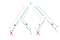Figure 5. Quantum coin — The two paths ultimately leading to heads have opposite and equal values cancelling each other while the two paths ultimately leading to tails add up reinforcing each other. This happens only when the box is not opened after first shake.
• But one may ask — how can branches of the tree cancel each other? When we played the game even with the quantum coin, the times we observed the coin after first shake clearly showed that each game run only traversed one path of the tree. That is, regardless of what we started with (heads or tails), we always took one of four paths in each run — HH, HT,TH,TT. So how can these paths cancel each other, when each path is different for each run?

This explanation was created based on Scott Aaronson’s blog. There is a book that is largely based on his blog Quantum Computing since Democritus: Scott Aaronson: Amazon.com: Books

For more details on what kind of “shaking operation” is performed on quantum coin etc, notes at the end of the answer to a related question may help How have physicists developed a mathematical model describing quantum phenomenon if we don’t really know what it means?

Originally published at www.quora.com.

Machine learning practitioner